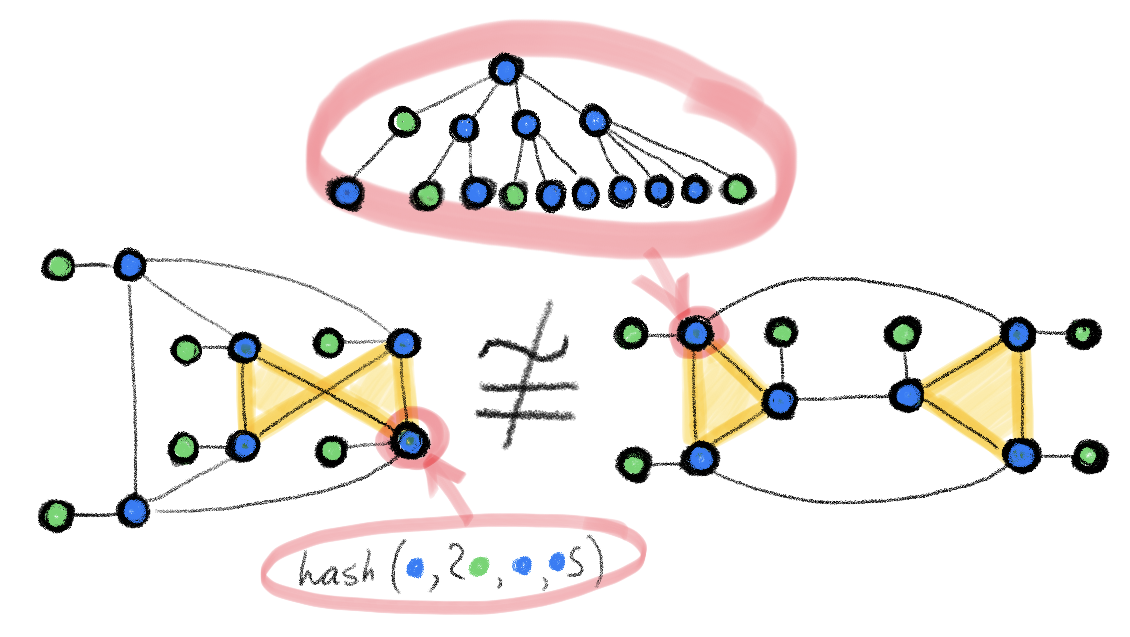# Expressive power of graph neural networks and the Weisfeiler-Lehman test

0

## Expressive power of graph neural networks and the Weisfeiler-Lehman test

### Expressive power of graph neural networks and the Weisfeiler-Lehman test“Traditional feed-forward networks (multi-layer perceptrons) are known to be universal approximators: they can approximate any smooth function to any desired accuracy. For graph neural networks, which have emerged relatively recently, the representation properties are less understood. It often happens to observe in experiments that graph neural networks excel on some datasets but at the same time perform disappointingly on others. In order to get to the root of this behaviour, one has to answer the question: how powerful are graph neural networks?
One of the challenges is that graphs encountered in applications are combinations of continuous and discrete structures (node- and edge features and connectivity, respectively), and thus this question can be posed in different ways. One possible formulation is whether graph neural networks can distinguish between different types of graph structures…”

March 30, 2021
Subscribe
Notify of